# Toc chapter 1 srg

8. May 2021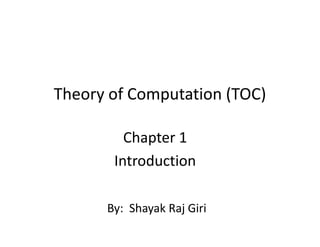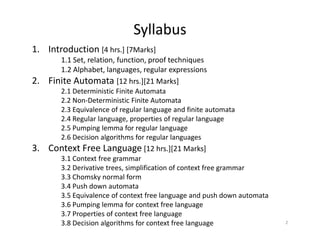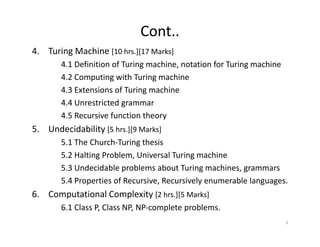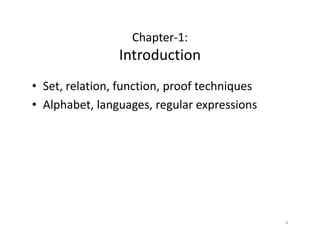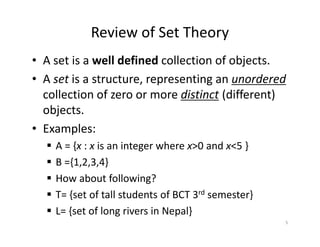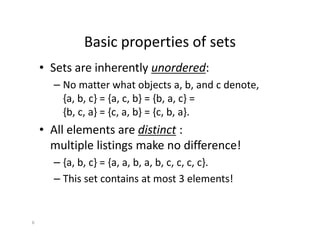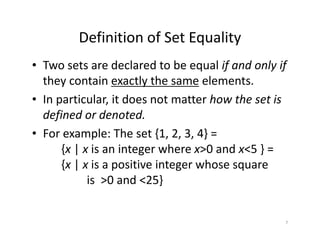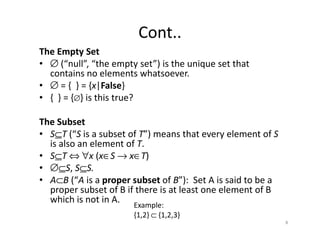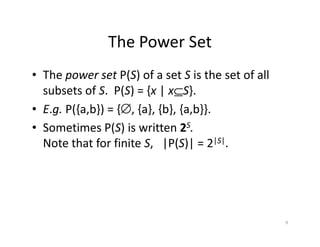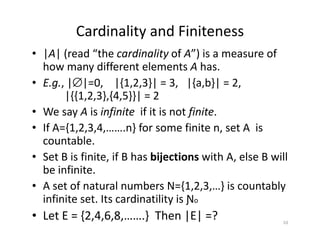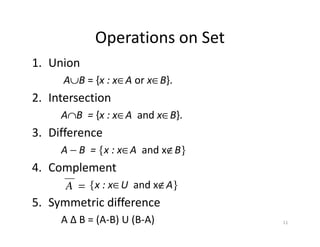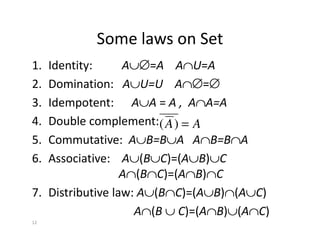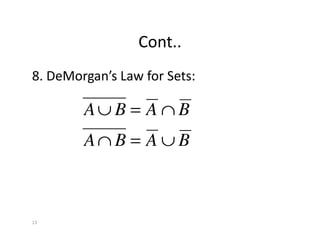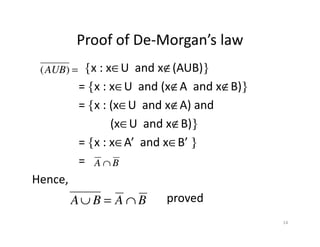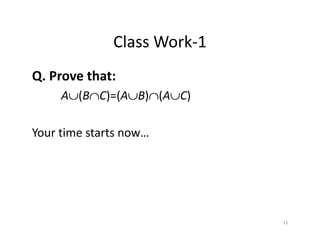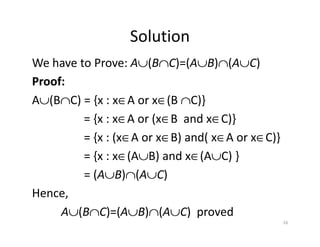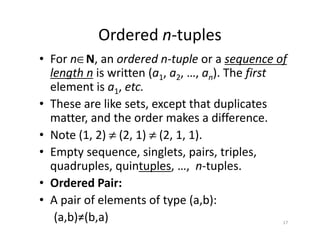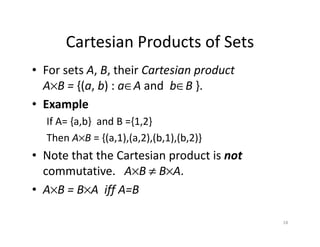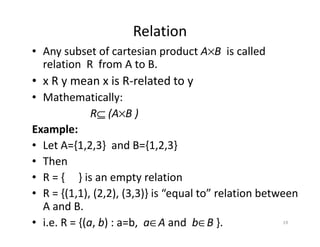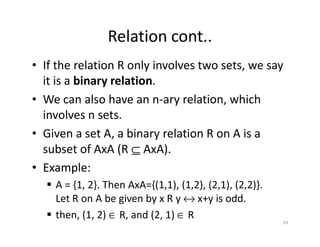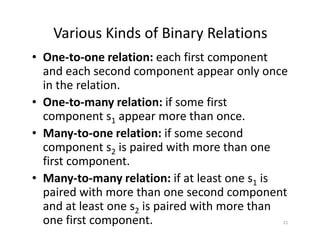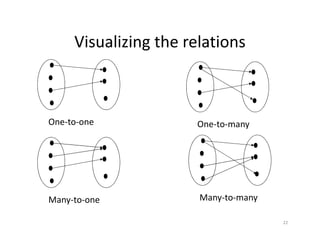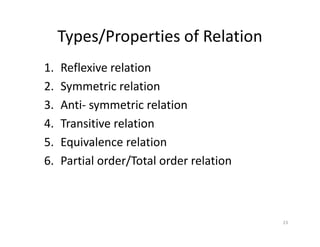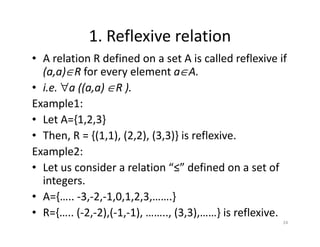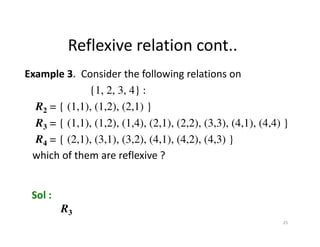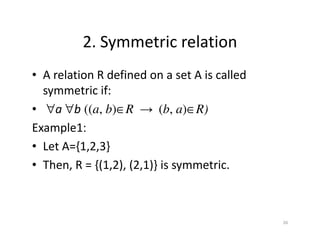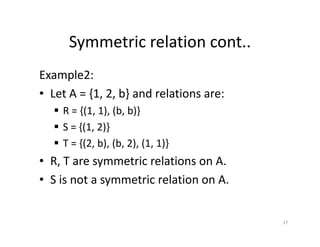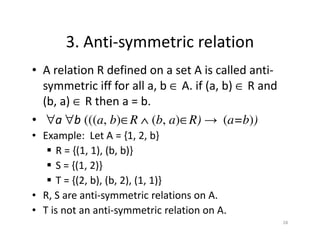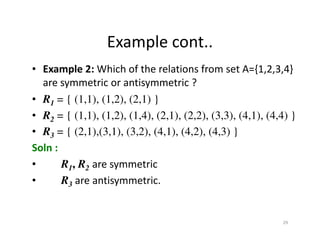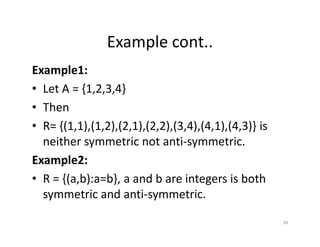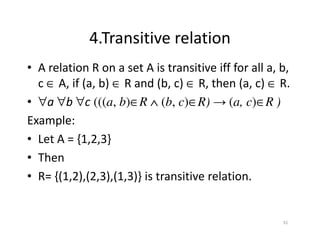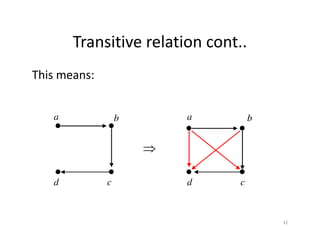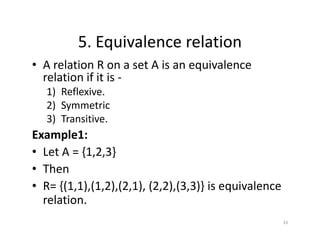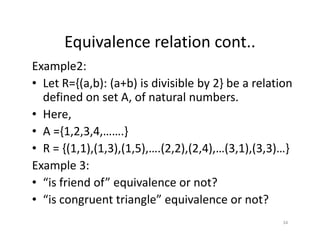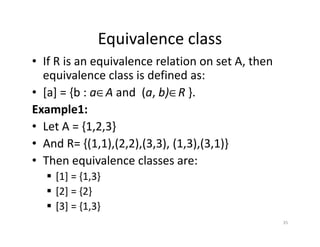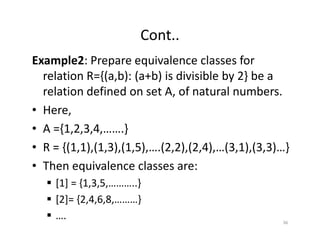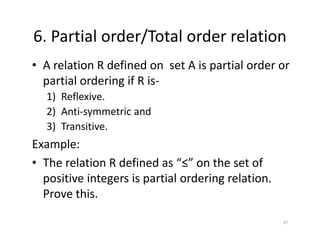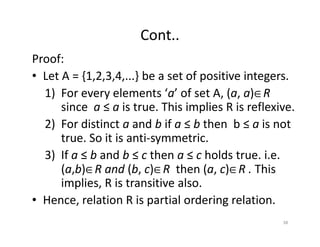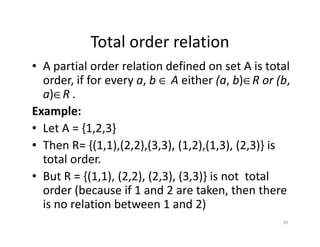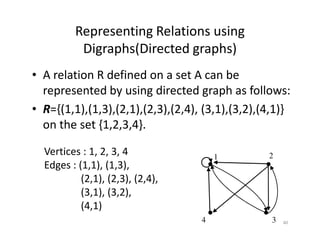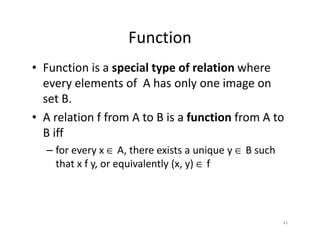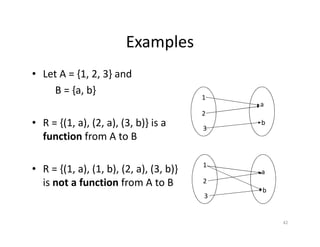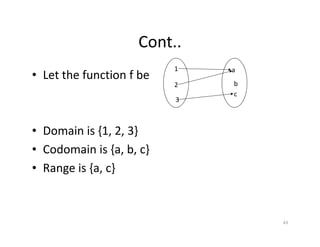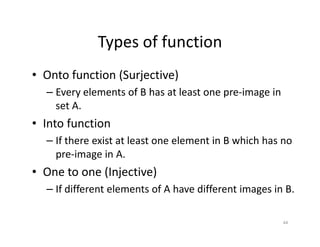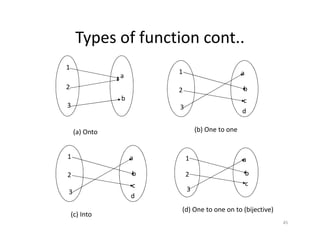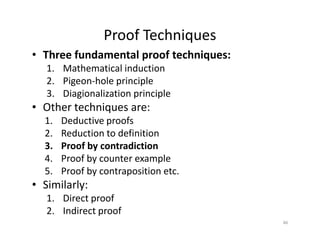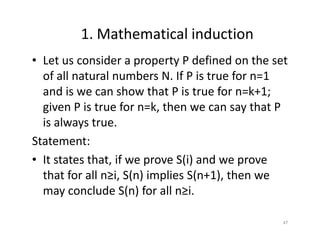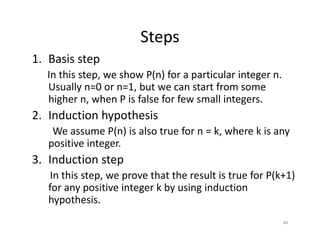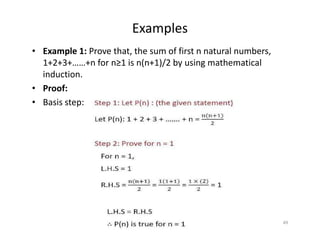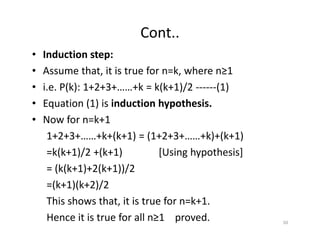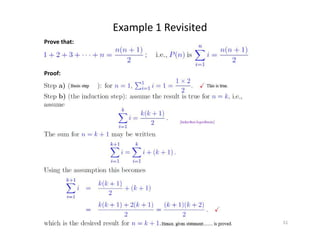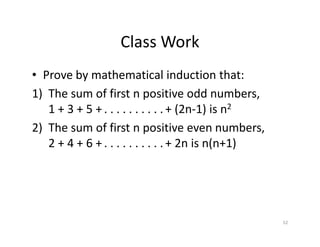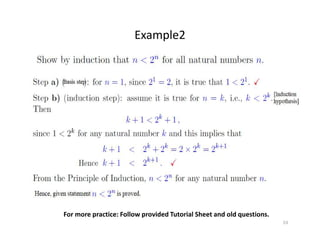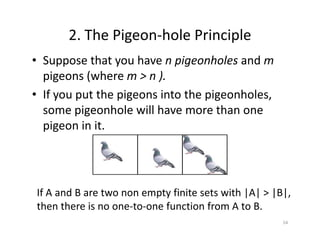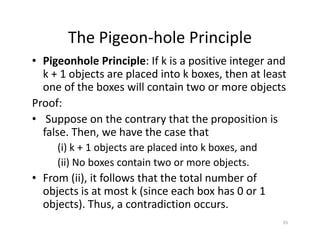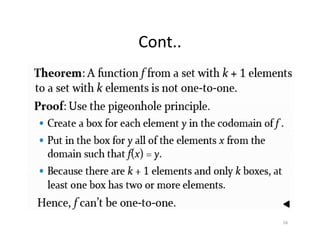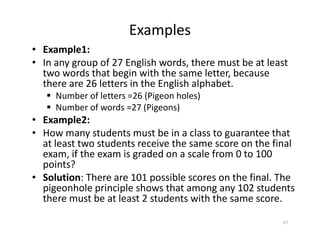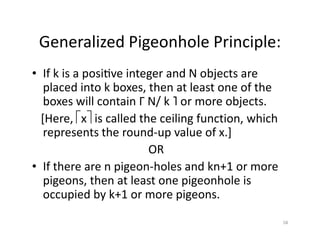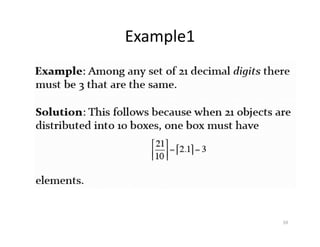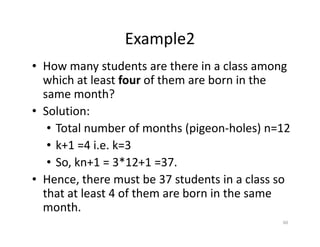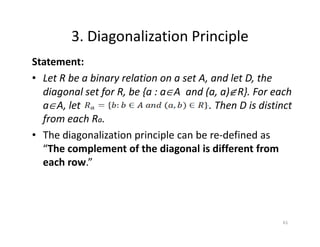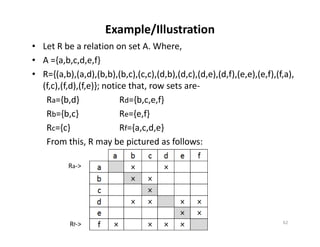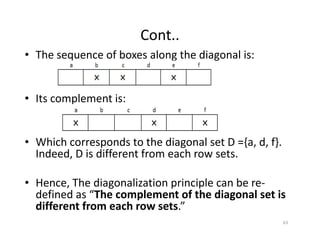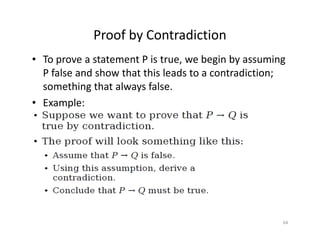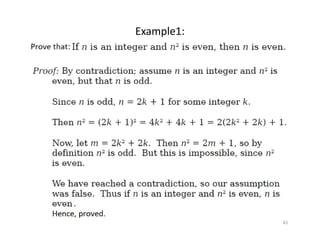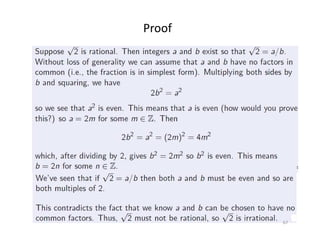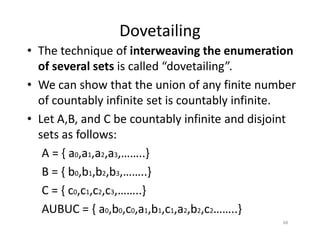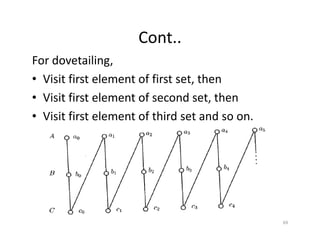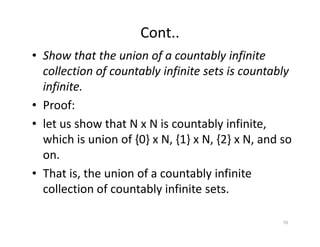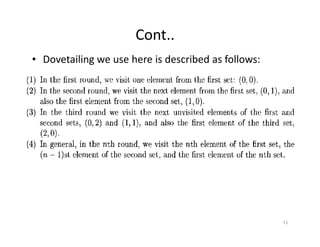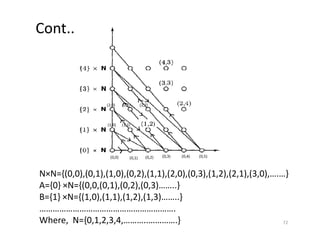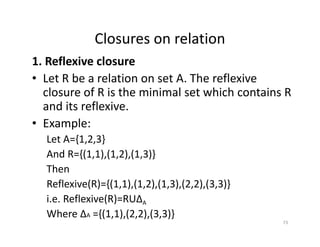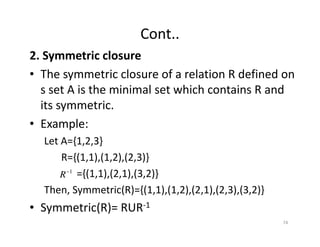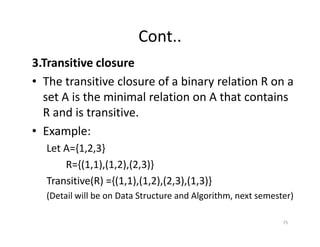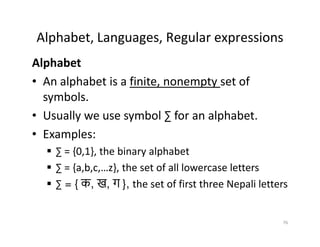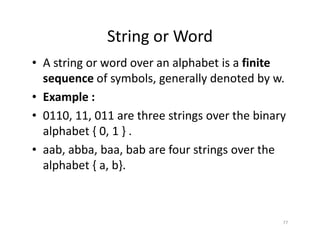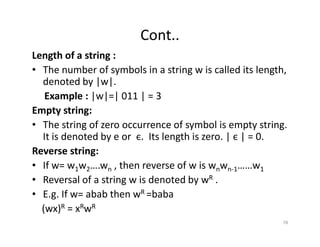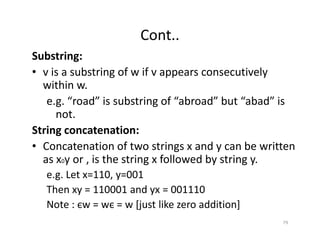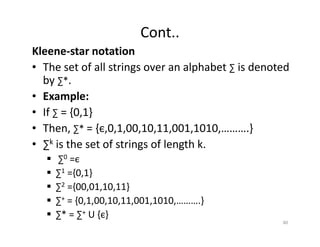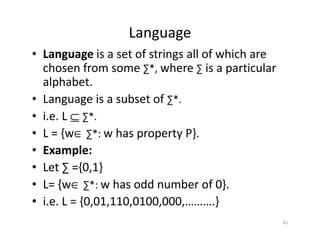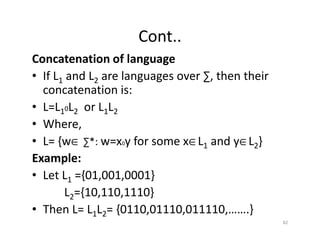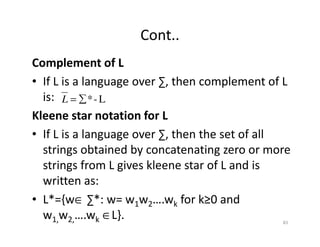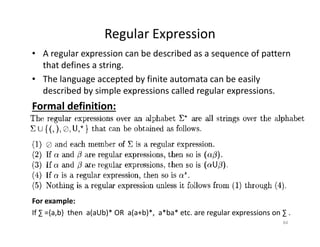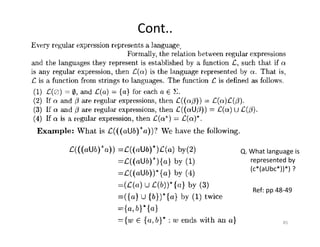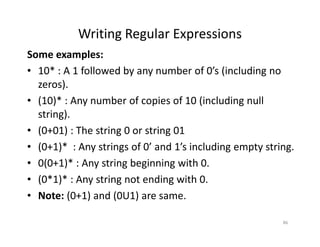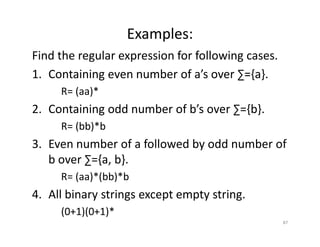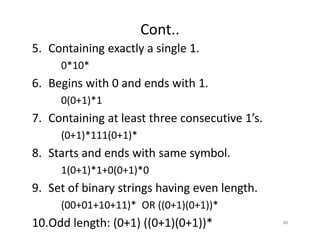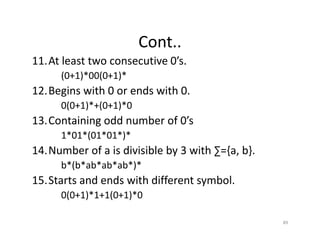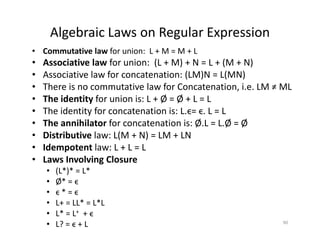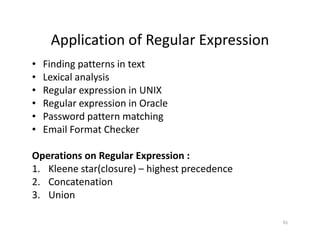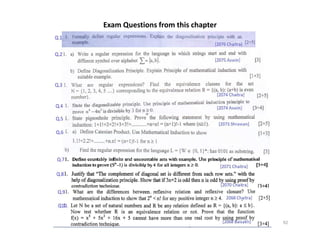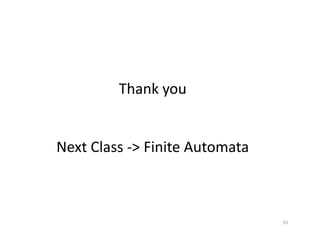1 von 93

### Toc chapter 1 srg

• 1. Theory of Computation (TOC) Chapter 1 Introduction By: Shayak Raj Giri
• 2. Syllabus 1. Introduction [4 hrs.] [7Marks] 1.1 Set, relation, function, proof techniques 1.2 Alphabet, languages, regular expressions 2. Finite Automata [12 hrs.][21 Marks] 2.1 Deterministic Finite Automata 2.2 Non‐Deterministic Finite Automata 2.3 Equivalence of regular language and finite automata 2.4 Regular language, properties of regular language 2.5 Pumping lemma for regular language 2.5 Pumping lemma for regular language 2.6 Decision algorithms for regular languages 3. Context Free Language [12 hrs.][21 Marks] 3.1 Context free grammar 3.2 Derivative trees, simplification of context free grammar 3.3 Chomsky normal form 3.4 Push down automata 3.5 Equivalence of context free language and push down automata 3.6 Pumping lemma for context free language 3.7 Properties of context free language 3.8 Decision algorithms for context free language 2
• 3. Cont.. 4. Turing Machine [10 hrs.][17 Marks] 4.1 Definition of Turing machine, notation for Turing machine 4.2 Computing with Turing machine 4.3 Extensions of Turing machine 4.4 Unrestricted grammar 4.5 Recursive function theory 5. Undecidability 5. Undecidability [5 hrs.][9 Marks] 5.1 The Church‐Turing thesis 5.2 Halting Problem, Universal Turing machine 5.3 Undecidable problems about Turing machines, grammars 5.4 Properties of Recursive, Recursively enumerable languages. 6. Computational Complexity [2 hrs.][5 Marks] 6.1 Class P, Class NP, NP‐complete problems. 3
• 4. Chapter‐1: Introduction • Set, relation, function, proof techniques • Alphabet, languages, regular expressions 4
• 5. Review of Set Theory • A set is a well defined collection of objects. • A set is a structure, representing an unordered collection of zero or more distinct (different) objects. • Examples: • Examples:  A = {x : x is an integer where x>0 and x<5 }  B ={1,2,3,4}  How about following?  T= {set of tall students of BCT 3rd semester}  L= {set of long rivers in Nepal} 5
• 6. Basic properties of sets • Sets are inherently unordered: – No matter what objects a, b, and c denote, {a, b, c} = {a, c, b} = {b, a, c} = {b, c, a} = {c, a, b} = {c, b, a}. • All elements are distinct : 6 • All elements are distinct : multiple listings make no difference! – {a, b, c} = {a, a, b, a, b, c, c, c, c}. – This set contains at most 3 elements!
• 7. Definition of Set Equality • Two sets are declared to be equal if and only if they contain exactly the same elements. • In particular, it does not matter how the set is defined or denoted. • For example: The set {1, 2, 3, 4} = {x | x is an integer where x>0 and x<5 } = {x | x is a positive integer whose square is >0 and <25} 7
• 8. Cont.. The Empty Set • ∅ (“null”, “the empty set”) is the unique set that contains no elements whatsoever. • ∅ = { } = {x|False} • { } = {∅} is this true? The Subset The Subset • S⊆T (“S is a subset of T”) means that every element of S is also an element of T. • S⊆T ⇔ ∀x (x∈S → x∈T) • ∅⊆S, S⊆S. • A⊂B (“A is a proper subset of B”): Set A is said to be a proper subset of B if there is at least one element of B which is not in A. Example: {1,2} ⊂ {1,2,3} 8
• 9. The Power Set • The power set P(S) of a set S is the set of all subsets of S. P(S) = {x | x⊆S}. • E.g. P({a,b}) = {∅, {a}, {b}, {a,b}}. • Sometimes P(S) is written 2S. • Sometimes P(S) is written 2S. Note that for finite S, |P(S)| = 2|S|. 9
• 10. Cardinality and Finiteness • |A| (read “the cardinality of A”) is a measure of how many different elements A has. • E.g., |∅|=0, |{1,2,3}| = 3, |{a,b}| = 2, |{{1,2,3},{4,5}}| = 2 • We say A is infinite if it is not finite. • If A={1,2,3,4,…….n} for some finite n, set A is • If A={1,2,3,4,…….n} for some finite n, set A is countable. • Set B is finite, if B has bijections with A, else B will be infinite. • A set of natural numbers N={1,2,3,…} is countably infinite set. Its cardinatility is Ɲo • Let E = {2,4,6,8,…….} Then |E| =? 10
• 11. Operations on Set 1. Union A∪B = {x : x∈A or x∈B}. 2. Intersection A∩B = {x : x∈A and x∈B}. 3. Difference A − B = {x : x∈A and x∉B} 4. Complement {x : x∈U and x∉A} 5. Symmetric difference A Δ B = (A‐B) U (B‐A) = A 11
• 12. Some laws on Set 1. Identity: A∪∅=A A∩U=A 2. Domination: A∪U=U A∩∅=∅ 3. Idempotent: A∪A = A , A∩A=A 4. Double complement: A A = ) ( 12 4. Double complement: 5. Commutative: A∪B=B∪A A∩B=B∩A 6. Associative: A∪(B∪C)=(A∪B)∪C A∩(B∩C)=(A∩B)∩C 7. Distributive law: A∪(B∩C)=(A∪B)∩(A∪C) A∩(B ∪ C)=(A∩B)∪(A∩C) A A = ) (
• 13. Cont.. 8. DeMorgan’s Law for Sets: B A B A ∩ = ∪ 13 B A B A ∪ = ∩
• 14. Proof of De‐Morgan’s law {x : x∈U and x∉(AUB)} = {x : x∈U and (x∉A and x∉B)} = {x : (x∈U and x∉A) and (x∈U and x∉B)} = ) (AUB (x∈U and x∉B)} = {x : x∈A’ and x∈B’ } = Hence, proved B A ∩ B A B A ∩ = ∪ 14
• 15. Class Work‐1 Q. Prove that: A∪(B∩C)=(A∪B)∩(A∪C) Your time starts now… 15
• 16. Solution We have to Prove: A∪(B∩C)=(A∪B)∩(A∪C) Proof: A∪(B∩C) = {x : x∈A or x∈(B ∩C)} = {x : x∈A or (x∈B and x∈C)} = {x : x∈A or (x∈B and x∈C)} = {x : (x∈A or x∈B) and( x∈A or x∈C)} = {x : x∈(A∪B) and x∈(A∪C) } = (A∪B)∩(A∪C) Hence, A∪(B∩C)=(A∪B)∩(A∪C) proved 16
• 17. Ordered n‐tuples • For n∈N, an ordered n-tuple or a sequence of length n is written (a1, a2, …, an). The first element is a1, etc. • These are like sets, except that duplicates matter, and the order makes a difference. matter, and the order makes a difference. • Note (1, 2) ≠ (2, 1) ≠ (2, 1, 1). • Empty sequence, singlets, pairs, triples, quadruples, quintuples, …, n‐tuples. • Ordered Pair: • A pair of elements of type (a,b): (a,b)≠(b,a) 17
• 18. Cartesian Products of Sets • For sets A, B, their Cartesian product A×B = {(a, b) : a∈A and b∈B }. • Example If A= {a,b} and B ={1,2} If A= {a,b} and B ={1,2} Then A×B = {(a,1),(a,2),(b,1),(b,2)} • Note that the Cartesian product is not commutative. A×B ≠ B×A. • A×B = B×A iff A=B 18
• 19. Relation • Any subset of cartesian product A×B is called relation R from A to B. • x R y mean x is R‐related to y • Mathematically: R⊆ (A×B ) Example: Example: • Let A={1,2,3} and B={1,2,3} • Then • R = { } is an empty relation • R = {(1,1), (2,2), (3,3)} is “equal to” relation between A and B. • i.e. R = {(a, b) : a=b, a∈A and b∈B }. 19
• 20. Relation cont.. • If the relation R only involves two sets, we say it is a binary relation. • We can also have an n‐ary relation, which involves n sets. • Given a set A, a binary relation R on A is a subset of AxA (R ⊆ AxA). • Example:  A = {1, 2}. Then AxA={(1,1), (1,2), (2,1), (2,2)}. Let R on A be given by x R y ↔ x+y is odd.  then, (1, 2) ∈ R, and (2, 1) ∈ R 20
• 21. Various Kinds of Binary Relations • One-to-one relation: each first component and each second component appear only once in the relation. • One-to-many relation: if some first component s1 appear more than once. component s1 appear more than once. • Many-to-one relation: if some second component s2 is paired with more than one first component. • Many-to-many relation: if at least one s1 is paired with more than one second component and at least one s2 is paired with more than one first component. 21
• 22. Visualizing the relations One‐to‐one One‐to‐many One‐to‐one One‐to‐many Many‐to‐one Many‐to‐many 22
• 23. Types/Properties of Relation 1. Reflexive relation 2. Symmetric relation 3. Anti‐ symmetric relation 4. Transitive relation 4. Transitive relation 5. Equivalence relation 6. Partial order/Total order relation 23
• 24. 1. Reflexive relation • A relation R defined on a set A is called reflexive if (a,a)∈R for every element a∈A. • i.e. ∀a ((a,a) ∈R ). Example1: • Let A={1,2,3} • Let A={1,2,3} • Then, R = {(1,1), (2,2), (3,3)} is reflexive. Example2: • Let us consider a relation “≤” defined on a set of integers. • A={….. ‐3,‐2,‐1,0,1,2,3,…….} • R={….. (‐2,‐2),(‐1,‐1), …….., (3,3),……} is reflexive. 24
• 25. Example 3. Consider the following relations on {1, 2, 3, 4} : R2 = { (1,1), (1,2), (2,1) } R3 = { (1,1), (1,2), (1,4), (2,1), (2,2), (3,3), (4,1), (4,4) } Reflexive relation cont.. R3 = { (1,1), (1,2), (1,4), (2,1), (2,2), (3,3), (4,1), (4,4) } R4 = { (2,1), (3,1), (3,2), (4,1), (4,2), (4,3) } which of them are reflexive ? Sol : R3 25
• 26. 2. Symmetric relation • A relation R defined on a set A is called symmetric if: • ∀a ∀b ((a, b)∈R → (b, a)∈R) Example1: Example1: • Let A={1,2,3} • Then, R = {(1,2), (2,1)} is symmetric. 26
• 27. Symmetric relation cont.. Example2: • Let A = {1, 2, b} and relations are:  R = {(1, 1), (b, b)}  S = {(1, 2)}  S = {(1, 2)}  T = {(2, b), (b, 2), (1, 1)} • R, T are symmetric relations on A. • S is not a symmetric relation on A. 27
• 28. 3. Anti‐symmetric relation • A relation R defined on a set A is called anti‐ symmetric iff for all a, b ∈ A. if (a, b) ∈ R and (b, a) ∈ R then a = b. • ∀a ∀b (((a, b)∈R ∧ (b, a)∈R) → (a=b)) • ∀a ∀b (((a, b)∈R ∧ (b, a)∈R) → (a=b)) • Example: Let A = {1, 2, b}  R = {(1, 1), (b, b)}  S = {(1, 2)}  T = {(2, b), (b, 2), (1, 1)} • R, S are anti‐symmetric relations on A. • T is not an anti‐symmetric relation on A. 28
• 29. Example cont.. • Example 2: Which of the relations from set A={1,2,3,4} are symmetric or antisymmetric ? • R1 = { (1,1), (1,2), (2,1) } • R2 = { (1,1), (1,2), (1,4), (2,1), (2,2), (3,3), (4,1), (4,4) } • R3 = { (2,1),(3,1), (3,2), (4,1), (4,2), (4,3) } Soln : • R1, R2 are symmetric • R3 are antisymmetric. 29
• 30. Example cont.. Example1: • Let A = {1,2,3,4} • Then • R= {(1,1),(1,2),(2,1),(2,2),(3,4),(4,1),(4,3)} is • R= {(1,1),(1,2),(2,1),(2,2),(3,4),(4,1),(4,3)} is neither symmetric not anti‐symmetric. Example2: • R = {(a,b):a=b}, a and b are integers is both symmetric and anti‐symmetric. 30
• 31. 4.Transitive relation • A relation R on a set A is transitive iff for all a, b, c ∈ A, if (a, b) ∈ R and (b, c) ∈ R, then (a, c) ∈ R. • ∀a ∀b ∀c (((a, b)∈R ∧ (b, c)∈R) → (a, c)∈R ) Example: Example: • Let A = {1,2,3} • Then • R= {(1,2),(2,3),(1,3)} is transitive relation. 31
• 32. Transitive relation cont.. This means: a b a b  d c d c 32
• 33. 5. Equivalence relation • A relation R on a set A is an equivalence relation if it is ‐ 1) Reflexive. 2) Symmetric 3) Transitive. 3) Transitive. Example1: • Let A = {1,2,3} • Then • R= {(1,1),(1,2),(2,1), (2,2),(3,3)} is equivalence relation. 33
• 34. Equivalence relation cont.. Example2: • Let R={(a,b): (a+b) is divisible by 2} be a relation defined on set A, of natural numbers. • Here, A ={1,2,3,4,…….} • A ={1,2,3,4,…….} • R = {(1,1),(1,3),(1,5),….(2,2),(2,4),…(3,1),(3,3)…} Example 3: • “is friend of” equivalence or not? • “is congruent triangle” equivalence or not? 34
• 35. Equivalence class • If R is an equivalence relation on set A, then equivalence class is defined as: • [a] = {b : a∈A and (a, b)∈R }. Example1: • Let A = {1,2,3} • Let A = {1,2,3} • And R= {(1,1),(2,2),(3,3), (1,3),(3,1)} • Then equivalence classes are:   = {1,3}   = {2}   = {1,3} 35
• 36. Cont.. Example2: Prepare equivalence classes for relation R={(a,b): (a+b) is divisible by 2} be a relation defined on set A, of natural numbers. • Here, • A ={1,2,3,4,…….} • A ={1,2,3,4,…….} • R = {(1,1),(1,3),(1,5),….(2,2),(2,4),…(3,1),(3,3)…} • Then equivalence classes are:   = {1,3,5,………..}  = {2,4,6,8,………}  …. 36
• 37. 6. Partial order/Total order relation • A relation R defined on set A is partial order or partial ordering if R is‐ 1) Reflexive. 2) Anti‐symmetric and 3) Transitive. 3) Transitive. Example: • The relation R defined as “≤” on the set of positive integers is partial ordering relation. Prove this. 37
• 38. Cont.. Proof: • Let A = {1,2,3,4,...} be a set of positive integers. 1) For every elements ‘a’ of set A, (a, a)∈R since a ≤ a is true. This implies R is reflexive. 2) For distinct a and b if a ≤ b then b ≤ a is not 2) For distinct a and b if a ≤ b then b ≤ a is not true. So it is anti‐symmetric. 3) If a ≤ b and b ≤ c then a ≤ c holds true. i.e. (a,b)∈R and (b, c)∈R then (a, c)∈R . This implies, R is transitive also. • Hence, relation R is partial ordering relation. 38
• 39. Total order relation • A partial order relation defined on set A is total order, if for every a, b ∈ A either (a, b)∈R or (b, a)∈R . Example: • Let A = {1,2,3} • Let A = {1,2,3} • Then R= {(1,1),(2,2),(3,3), (1,2),(1,3), (2,3)} is total order. • But R = {(1,1), (2,2), (2,3), (3,3)} is not total order (because if 1 and 2 are taken, then there is no relation between 1 and 2) 39
• 40. Representing Relations using Digraphs(Directed graphs) • A relation R defined on a set A can be represented by using directed graph as follows: • R={(1,1),(1,3),(2,1),(2,3),(2,4), (3,1),(3,2),(4,1)} on the set {1,2,3,4}. on the set {1,2,3,4}. Vertices : 1, 2, 3, 4 Edges : (1,1), (1,3), (2,1), (2,3), (2,4), (3,1), (3,2), (4,1) 1 2 4 3 40
• 41. Function • Function is a special type of relation where every elements of A has only one image on set B. • A relation f from A to B is a function from A to • A relation f from A to B is a function from A to B iff – for every x ∈ A, there exists a unique y ∈ B such that x f y, or equivalently (x, y) ∈ f 41
• 42. Examples • Let A = {1, 2, 3} and B = {a, b} • R = {(1, a), (2, a), (3, b)} is a 1 2 a b • R = {(1, a), (2, a), (3, b)} is a function from A to B • R = {(1, a), (1, b), (2, a), (3, b)} is not a function from A to B 1 2 3 a b 3 b 42
• 43. Cont.. • Let the function f be • Domain is {1, 2, 3} 1 2 3 a b c • Domain is {1, 2, 3} • Codomain is {a, b, c} • Range is {a, c} 43
• 44. Types of function • Onto function (Surjective) – Every elements of B has at least one pre‐image in set A. • Into function • Into function – If there exist at least one element in B which has no pre‐image in A. • One to one (Injective) – If different elements of A have different images in B. 44
• 45. Types of function cont.. 1 2 3 a b 1 2 3 a b c d 1 2 3 a b c (a) Onto (b) One to one 1 2 3 a b c d (c) Into (d) One to one on to (bijective) 45
• 46. Proof Techniques • Three fundamental proof techniques: 1. Mathematical induction 2. Pigeon‐hole principle 3. Diagionalization principle • Other techniques are: 1. Deductive proofs 1. Deductive proofs 2. Reduction to definition 3. Proof by contradiction 4. Proof by counter example 5. Proof by contraposition etc. • Similarly: 1. Direct proof 2. Indirect proof 46
• 47. 1. Mathematical induction • Let us consider a property P defined on the set of all natural numbers N. If P is true for n=1 and is we can show that P is true for n=k+1; given P is true for n=k, then we can say that P is always true. is always true. Statement: • It states that, if we prove S(i) and we prove that for all n≥i, S(n) implies S(n+1), then we may conclude S(n) for all n≥i. 47
• 48. Steps 1. Basis step In this step, we show P(n) for a particular integer n. Usually n=0 or n=1, but we can start from some higher n, when P is false for few small integers. 2. Induction hypothesis We assume P(n) is also true for n = k, where k is any positive integer. 3. Induction step In this step, we prove that the result is true for P(k+1) for any positive integer k by using induction hypothesis. 48
• 49. Examples • Example 1: Prove that, the sum of first n natural numbers, 1+2+3+……+n for n≥1 is n(n+1)/2 by using mathematical induction. • Proof: • Basis step: 49
• 50. Cont.. • Induction step: • Assume that, it is true for n=k, where n≥1 • i.e. P(k): 1+2+3+……+k = k(k+1)/2 ‐‐‐‐‐‐(1) • Equation (1) is induction hypothesis. • Now for n=k+1 • Now for n=k+1 1+2+3+……+k+(k+1) = (1+2+3+……+k)+(k+1) =k(k+1)/2 +(k+1) [Using hypothesis] = (k(k+1)+2(k+1))/2 =(k+1)(k+2)/2 This shows that, it is true for n=k+1. Hence it is true for all n≥1 proved. 50
• 51. Example 1 Revisited Prove that: Proof: 51
• 52. Class Work • Prove by mathematical induction that: 1) The sum of first n positive odd numbers, 1 + 3 + 5 + . . . . . . . . . . + (2n‐1) is n2 2) The sum of first n positive even numbers, 2) The sum of first n positive even numbers, 2 + 4 + 6 + . . . . . . . . . . + 2n is n(n+1) 52
• 53. Example2 For more practice: Follow provided Tutorial Sheet and old questions. 53
• 54. 2. The Pigeon‐hole Principle • Suppose that you have n pigeonholes and m pigeons (where m > n ). • If you put the pigeons into the pigeonholes, some pigeonhole will have more than one pigeon in it. pigeon in it. If A and B are two non empty finite sets with |A| > |B|, then there is no one‐to‐one function from A to B. 54
• 55. The Pigeon‐hole Principle • Pigeonhole Principle: If k is a positive integer and k + 1 objects are placed into k boxes, then at least one of the boxes will contain two or more objects Proof: • Suppose on the contrary that the proposition is • Suppose on the contrary that the proposition is false. Then, we have the case that (i) k + 1 objects are placed into k boxes, and (ii) No boxes contain two or more objects. • From (ii), it follows that the total number of objects is at most k (since each box has 0 or 1 objects). Thus, a contradiction occurs. 55
• 57. Examples • Example1: • In any group of 27 English words, there must be at least two words that begin with the same letter, because there are 26 letters in the English alphabet.  Number of letters =26 (Pigeon holes)  Number of words =27 (Pigeons) • Example2: • Example2: • How many students must be in a class to guarantee that at least two students receive the same score on the final exam, if the exam is graded on a scale from 0 to 100 points? • Solution: There are 101 possible scores on the final. The pigeonhole principle shows that among any 102 students there must be at least 2 students with the same score. 57
• 58. Generalized Pigeonhole Principle: • If k is a posiYve integer and N objects are placed into k boxes, then at least one of the boxes will contain Г N/ k ˥ or more objects. [Here, x is called the ceiling function, which [Here, x is called the ceiling function, which represents the round‐up value of x.] OR • If there are n pigeon‐holes and kn+1 or more pigeons, then at least one pigeonhole is occupied by k+1 or more pigeons. 58
• 60. Example2 • How many students are there in a class among which at least four of them are born in the same month? • Solution: • Total number of months (pigeon‐holes) n=12 • Total number of months (pigeon‐holes) n=12 • k+1 =4 i.e. k=3 • So, kn+1 = 3*12+1 =37. • Hence, there must be 37 students in a class so that at least 4 of them are born in the same month. 60
• 61. 3. Diagonalization Principle Statement: • Let R be a binary relation on a set A, and let D, the diagonal set for R, be {a : a∈A and (a, a)∉R}. For each a∈A, let Ra = { b: b∈A and (a, b)∈R}. Then D is distinct from each Ra. from each Ra. • The diagonalization principle can be re‐defined as “The complement of the diagonal is different from each row.” 61
• 62. Example/Illustration • Let R be a relation on set A. Where, • A ={a,b,c,d,e,f} • R={(a,b),(a,d),(b,b),(b,c),(c,c),(d,b),(d,c),(d,e),(d,f),(e,e),(e,f),(f,a), (f,c),(f,d),(f,e)}; notice that, row sets are‐ Ra={b,d} Rd={b,c,e,f} Rb={b,c} Re={e,f} Rc={c} Rf={a,c,d,e} Rc={c} Rf={a,c,d,e} From this, R may be pictured as follows: Ra‐> Rf‐> 62
• 63. Cont.. • The sequence of boxes along the diagonal is: • Its complement is: • Which corresponds to the diagonal set D ={a, d, f}. Indeed, D is different from each row sets. • Hence, The diagonalization principle can be re‐ defined as “The complement of the diagonal set is different from each row sets.” 63
• 64. Proof by Contradiction • To prove a statement P is true, we begin by assuming P false and show that this leads to a contradiction; something that always false. • Example: 64
• 66. Example2 Prove that: √2 is irrational. 66
• 68. Dovetailing • The technique of interweaving the enumeration of several sets is called “dovetailing”. • We can show that the union of any finite number of countably infinite set is countably infinite. • Let A,B, and C be countably infinite and disjoint • Let A,B, and C be countably infinite and disjoint sets as follows: A = { a0,a1,a2,a3,……..} B = { b0,b1,b2,b3,……..} C = { c0,c1,c2,c3,……..} AUBUC = { a0,b0,c0,a1,b1,c1,a2,b2,c2……..} 68
• 69. Cont.. For dovetailing, • Visit first element of first set, then • Visit first element of second set, then • Visit first element of third set and so on. Visit first element of third set and so on. 69
• 70. Cont.. • Show that the union of a countably infinite collection of countably infinite sets is countably infinite. • Proof: • let us show that N x N is countably infinite, • let us show that N x N is countably infinite, which is union of {0} x N, {1} x N, {2} x N, and so on. • That is, the union of a countably infinite collection of countably infinite sets. 70
• 71. Cont.. • Dovetailing we use here is described as follows: 71
• 73. Closures on relation 1. Reflexive closure • Let R be a relation on set A. The reflexive closure of R is the minimal set which contains R and its reflexive. • Example: • Example: Let A={1,2,3} And R={(1,1),(1,2),(1,3)} Then Reflexive(R)={(1,1),(1,2),(1,3),(2,2),(3,3)} i.e. Reflexive(R)=RUΔA Where ΔA ={(1,1),(2,2),(3,3)} 73
• 74. Cont.. 2. Symmetric closure • The symmetric closure of a relation R defined on s set A is the minimal set which contains R and its symmetric. • Example: • Example: Let A={1,2,3} R={(1,1),(1,2),(2,3)} ={(1,1),(2,1),(3,2)} Then, Symmetric(R)={(1,1),(1,2),(2,1),(2,3),(3,2)} • Symmetric(R)= RUR‐1 1 − R 74
• 75. Cont.. 3.Transitive closure • The transitive closure of a binary relation R on a set A is the minimal relation on A that contains R and is transitive. • Example: • Example: Let A={1,2,3} R={(1,1),(1,2),(2,3)} Transitive(R) ={(1,1),(1,2),(2,3),(1,3)} (Detail will be on Data Structure and Algorithm, next semester) 75
• 76. Alphabet, Languages, Regular expressions Alphabet • An alphabet is a finite, nonempty set of symbols. • Usually we use symbol ∑ for an alphabet. • Examples:  ∑ = {0,1}, the binary alphabet  ∑ = {a,b,c,…z}, the set of all lowercase letters  ∑ = { क, ख, ग }, the set of first three Nepali letters 76
• 77. String or Word • A string or word over an alphabet is a finite sequence of symbols, generally denoted by w. • Example : • 0110, 11, 011 are three strings over the binary alphabet { 0, 1 } . alphabet { 0, 1 } . • aab, abba, baa, bab are four strings over the alphabet { a, b}. 77
• 78. Cont.. Length of a string : • The number of symbols in a string w is called its length, denoted by |w|. Example : |w|=| 011 | = 3 Empty string: • The string of zero occurrence of symbol is empty string. • The string of zero occurrence of symbol is empty string. It is denoted by e or є. Its length is zero. | є | = 0. Reverse string: • If w= w1w2….wn , then reverse of w is wnwn‐1……w1 • Reversal of a string w is denoted by wR . • E.g. If w= abab then wR =baba (wx)R = xRwR 78
• 79. Cont.. Substring: • v is a substring of w if v appears consecutively within w. e.g. “road” is substring of “abroad” but “abad” is not. String concatenation: String concatenation: • Concatenation of two strings x and y can be written as x0y or , is the string x followed by string y. e.g. Let x=110, y=001 Then xy = 110001 and yx = 001110 Note : єw = wє = w [just like zero addition] 79
• 80. Cont.. Kleene-star notation • The set of all strings over an alphabet ∑ is denoted by ∑*. • Example: • If ∑ = {0,1} • Then, ∑* = {є,0,1,00,10,11,001,1010,……….} • Then, ∑* = {є,0,1,00,10,11,001,1010,……….} • ∑k is the set of strings of length k.  ∑0 =є  ∑1 ={0,1}  ∑2 ={00,01,10,11}  ∑+ = {0,1,00,10,11,001,1010,……….}  ∑* = ∑+ U {є} 80
• 81. Language • Language is a set of strings all of which are chosen from some ∑*, where ∑ is a particular alphabet. • Language is a subset of ∑*. • i.e. L ⊆ ∑*. i.e. L • L = {w∈ ∑*: w has property P}. • Example: • Let ∑ ={0,1} • L= {w∈ ∑*: w has odd number of 0}. • i.e. L = {0,01,110,0100,000,……….} 81
• 82. Cont.. Concatenation of language • If L1 and L2 are languages over ∑, then their concatenation is: • L=L10L2 or L1L2 • Where, • Where, • L= {w∈ ∑*: w=x0y for some x∈L1 and y∈L2} Example: • Let L1 ={01,001,0001} L2={10,110,1110} • Then L= L1L2= {0110,01110,011110,…….} 82
• 83. Cont.. Complement of L • If L is a language over ∑, then complement of L is: Kleene star notation for L L - *  = L • If L is a language over ∑, then the set of all strings obtained by concatenating zero or more strings from L gives kleene star of L and is written as: • L*={w∈ ∑*: w= w1w2….wk for k≥0 and w1,w2,….wk ∈L}. 83
• 84. Regular Expression • A regular expression can be described as a sequence of pattern that defines a string. • The language accepted by finite automata can be easily described by simple expressions called regular expressions. Formal definition: For example: If ∑ ={a,b} then a(aUb)* OR a(a+b)*, a*ba* etc. are regular expressions on ∑ . 84
• 85. Cont.. Q. What language is represented by (c*(aUbc*))*) ? Ref: pp 48‐49 85
• 86. Writing Regular Expressions Some examples: • 10* : A 1 followed by any number of 0’s (including no zeros). • (10)* : Any number of copies of 10 (including null string). (0+01) : The string 0 or string 01 • (0+01) : The string 0 or string 01 • (0+1)* : Any strings of 0’ and 1’s including empty string. • 0(0+1)* : Any string beginning with 0. • (0*1)* : Any string not ending with 0. • Note: (0+1) and (0U1) are same. 86
• 87. Examples: Find the regular expression for following cases. 1. Containing even number of a’s over ∑={a}. R= (aa)* 2. Containing odd number of b’s over ∑={b}. R= (bb)*b R= (bb)*b 3. Even number of a followed by odd number of b over ∑={a, b}. R= (aa)*(bb)*b 4. All binary strings except empty string. (0+1)(0+1)* 87
• 88. Cont.. 5. Containing exactly a single 1. 0*10* 6. Begins with 0 and ends with 1. 0(0+1)*1 7. Containing at least three consecutive 1’s. 7. Containing at least three consecutive 1’s. (0+1)*111(0+1)* 8. Starts and ends with same symbol. 1(0+1)*1+0(0+1)*0 9. Set of binary strings having even length. (00+01+10+11)* OR ((0+1)(0+1))* 10.Odd length: (0+1) ((0+1)(0+1))* 88
• 89. Cont.. 11.At least two consecutive 0’s. (0+1)*00(0+1)* 12.Begins with 0 or ends with 0. 0(0+1)*+(0+1)*0 13.Containing odd number of 0’s 13.Containing odd number of 0’s 1*01*(01*01*)* 14.Number of a is divisible by 3 with ∑={a, b}. b*(b*ab*ab*ab*)* 15.Starts and ends with different symbol. 0(0+1)*1+1(0+1)*0 89
• 90. Algebraic Laws on Regular Expression • Commutative law for union: L + M = M + L • Associative law for union: (L + M) + N = L + (M + N) • Associative law for concatenation: (LM)N = L(MN) • There is no commutative law for Concatenation, i.e. LM ≠ ML • The identity for union is: L + Ø = Ø + L = L • The identity for concatenation is: L.ϵ= ϵ. L = L • The annihilator for concatenation is: Ø.L = L.Ø = Ø • The annihilator for concatenation is: Ø.L = L.Ø = Ø • Distributive law: L(M + N) = LM + LN • Idempotent law: L + L = L • Laws Involving Closure • (L*)* = L* • Ø* = ϵ • ϵ * = ϵ • L+ = LL* = L*L • L* = L+ + ϵ • L? = ϵ + L 90
• 91. Application of Regular Expression • Finding patterns in text • Lexical analysis • Regular expression in UNIX • Regular expression in Oracle • Password pattern matching • Email Format Checker • Email Format Checker Operations on Regular Expression : 1. Kleene star(closure) – highest precedence 2. Concatenation 3. Union 91
• 92. Exam Questions from this chapter 92
• 93. Thank you Next Class ‐> Finite Automata 93